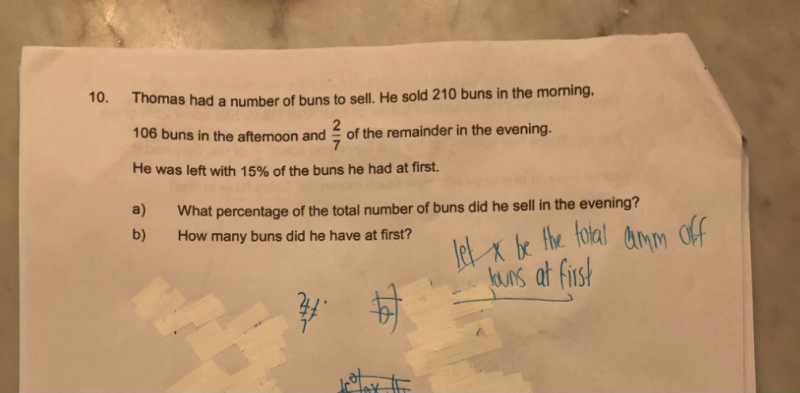# Question(a)  Morning  sold —> 210

Afternoon sold —> 106      => Total = 316 buns

sold   <—-left  15%–>

Night    [u][u] [——-5u——–]

5u -> 15%

u -> 15/5 = 3

Hence sold = 2u = 2x 3 = 6%

(b) 15 + 6 = 21   So morning + afternoon sold = 100-21 = 79%

79% -> 316

100% = (316/79) x 100 = 400

Hence number of buns he has at first = 400